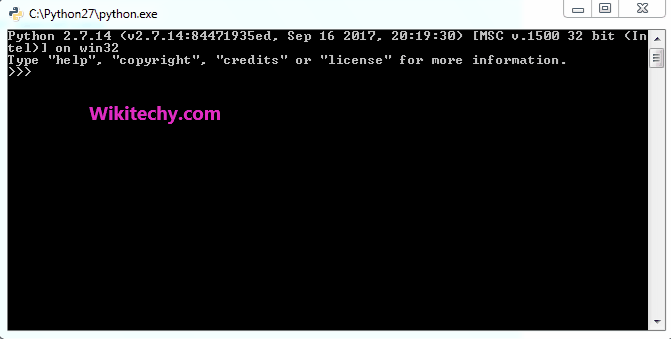# python tutorial - Python Iterator | Iterator Functions in Python - learn python - python programming

Python in its definition also allows some interesting and useful iterator functions for efficient looping and making execution of the code faster. There are many build-in iterators in the module “itertools“.
This module implements a number of iterator building blocks.
Some useful Iterators :

1. accumulate(iter, func) :- This iterator takes two arguments, iterable target and the function which would be followed at each iteration of value in target. If no function is passed, addition takes place by default.If the input iterable is empty, the output iterable will also be empty.

2. chain(iter1, iter2..) :- This function is used to print all the values in iterable targets one after another mentioned in its arguments.Learn Python - Python tutorial - python iterator function - Python examples - Python programs

python - Sample - python code :

python tutorial - Output :

```The sum after each iteration is : [1, 5, 10, 17]
The product after each iteration is : [1, 4, 20, 140]
All values in mentioned chain are : [1, 4, 5, 7, 1, 6, 5, 9, 8, 10, 5, 4]
```

3. chain.from_iterable() :- This function is implemented similarly as chain() but the argument here is a list of lists or any other iterable container.

4. compress(iter, selector) :- This iterator selectively picks the values to print from the passed container according to the boolean list value passed as other argument. The arguments corresponding to boolean true are printed else all are skipped.

python - Sample - python code :

python tutorial - Output :

```All values in mentioned chain are : [1, 4, 5, 7, 1, 6, 5, 9, 8, 10, 5, 4]
The compressed values in string are : ['W', 'E', 'Y']
```

5. dropwhile(func, seq) :- This iterator starts printing the characters only after the func. in argument returns false for the first time.

6. filterfalse(func, seq) :- As the name suggests, this iterator prints only values that return false for the passed function.

python - Sample - python code :

python tutorial - Output :

```The values after condition returns false : [5, 7, 8]
The values that return false to function are : [5, 7]
```

Wikitechy tutorial site provides you all the learn python , python code , python script , python windows , python mysql , django python , python django , python compiler , jython , python interpreter , what is python , python join , python input , python modules , python editor , python examples , python pdf , python documentation